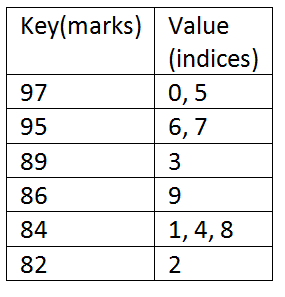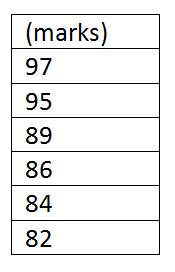# Toppers of Class

Toppers of the Class - is an interview coding problem came in the coding round of D.E.Shaw. Here, we are going to implement its solution along with example and algorithm.
Submitted by Radib Kar, on December 15, 2018

Problem statement:

There is a class of N students and the task is to find the top K marks-scorers. Write a program that will print the index of the toppers of the class which will be same as the index of the student in the input array (use 0-based indexing). First print the index of the students having highest marks then the students with second highest and so on. If there are more than one students having same marks then print their indices in ascending order.

Input Example:

Suppose k = 2 and the students having highest marks have indices 0 and 5 and students having second highest marks have indices 6 and 7 then output will be 0 5 6 7.

```    K=2
N=10
Marks are:
97 84 82 89 84 97 95 95 84 86
Output:
0 5 6 7, so there are four toppers for K=2
```

97 & 95 is to be considered. There are four students who has got this. Their indices are 0 5 6 7 (For a particular marks if there is more than one student, their indices needs to printed in ascending order).

Solution:

Data structure used:

1. Set (ordered in decreasing fashion)
2. Map (ordered in decreasing fashion)

Algorithm:

1. Need to store the marks in sorted way descending order.
2. The marks are key and we need to map student indices to the key value. While mapping indices needed to be mapped in ascending fashion.
3. Print indices for K keys ( marks value already sorted in decreasing fashion, thus top K keys are top K marks).

Implementation with the data structures used:

1. Declare records as a map.
```map<int, vector<int>, greater <int>> records;
```
map<> = ordered map usually ordered in ascending fashion as per key value greater <int> is used to order in descending fashion.
Here our key is integer type which maps to a vector of integer. Clearly the key is marks & which maps to a list of indices of students.
2. Declare numbers as a set.
```set<int, greater<int>> numbers;
```
set<> = ordered set usually ordered in ascending fashion as per element value greater <int> is used to order in descending fashion.
Here the elements are the marks which are stored in sorted descending fashion.
3. After completion of the input taking, both records&numbers are filled with datas as per mentioned previously.

Let's consider the above input example:

```    K=2
N=10
Marks are:
97 84 82 89 84 97 95 95 84 86
```

So after completion of input taking:

Records looks like:Numbers looks like:K=2, thus we need to print indices only for marks 97, 95

Thus the indices to be print are: 0 5 6 7

So to print:

```For i =0: K
Set iterator tonumbers.begin() //points to 97
Advance iterator by i; //to point at ith mark from the top
Print the vector list associated with the key (marks) pointed to.
END FOR
```

## C++ implementation for Toppers of Class

```#include <bits/stdc++.h>
using namespace std;

int main()
{
int n,k;
//n is no of students, k is the input K

cout<<"enter no of student\n";
scanf("%d",&n);

map<int,vector<int>,greater <int>> records;//declare records
set<int,greater<int>> numbers;//declare numbers

int no;
cout<<"enter the marks of the students\n";
for(int i=0;i<n;i++){
cin>>no;
//for key value build the vector list
records[no].push_back(i);
//to avoid duplicate
if(numbers.find(no)==numbers.end())
numbers.insert(no); //insert marks to set
}

cout<<"enter K\n";
cin>>k; //input K

cout<<"Toppers are: ";
//printing the indices
for(int i=0;i<k;i++){
auto ij=numbers.begin();
//printing the associated vector
for(auto it=records[*ij].begin();it!=records[*ij].end();it++){
printf("%d ",*it);
}
}

cout<<endl;

return 0;
}
```

Output

```enter no of student
10
enter the marks of the students
97 84 82 89 84 97 95 95 84 86
enter K
2
Toppers are: 0 5 6 7
```